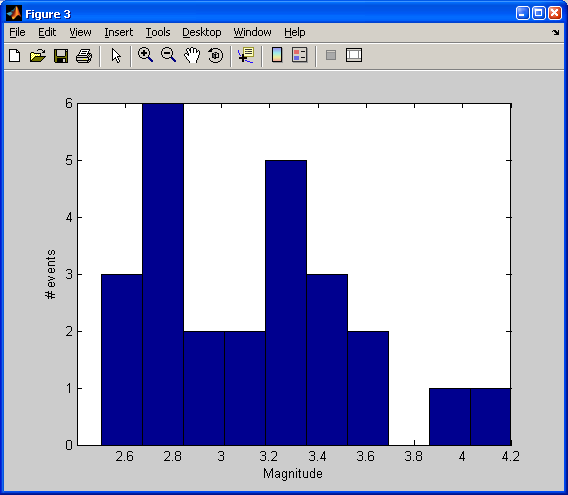# Graphical Interaction with Plots and Subsetting

## Quick reminder of Yellowstone

Yellowstone - Teton epicenter

## Using paths in Matlab to keep things tidy

### ginput, gtext, and find

Today's lecture will continue a discussion of tools for exploring your data.

#### Set up the example

Let's go back to our Yellowstone seismicity data.

I am going to load the data, set up a few variables, and then plot it:
```load('ystone_eqs_sort.txt','-ascii');
year = ystone_eqs_sort(:,1);
x_locations = ystone_eqs_sort(:,4);
y_locations = ystone_eqs_sort(:,3);
depth = ystone_eqs_sort(:,5);
magnitudes = ystone_eqs_sort(:,6);

figure(1)
clf
plot(x_locations,y_locations,'k.')
xlabel('longitude')
ylabel('latitude')
axis equal
```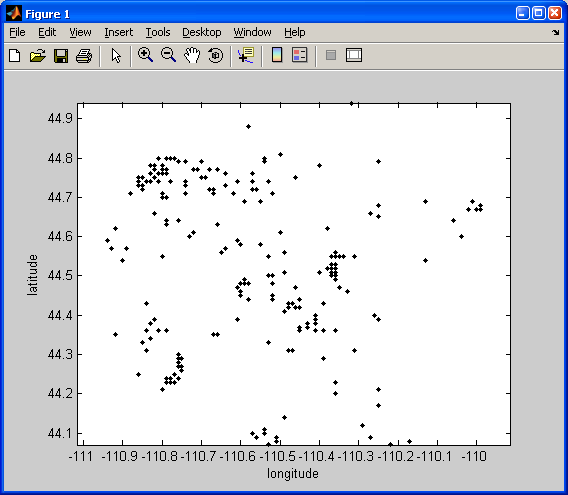#### Getting the locations of mouse clicks on a plot

Say you want to have the user select some portion of the data interactively using the mouse. You can get the coordinates of a user's clicks by using the Matlab command ginput.

I added this code to my script so that you can tell the user what to do:
`fprintf(1, 'click on lower left and upper right of the area of interest\n')`
Note that the
`\n`
means "add a new line after this."

NOTE: fprintf is a function that is used to write out text (to a file or the command window).

The "1" as the first argument in fprintf tells the function to print the text to the screen.

The script entry looks like this:
```[X,Y] = ginput(2);

xmin=min(X)
xmax=max(X)
ymin=min(Y)
ymax=max(Y)
```
The argument of 2 to ginput tells it to take two clicks. When you move the mouse over the figure, you will see that the cursor turns into a moving cross hair: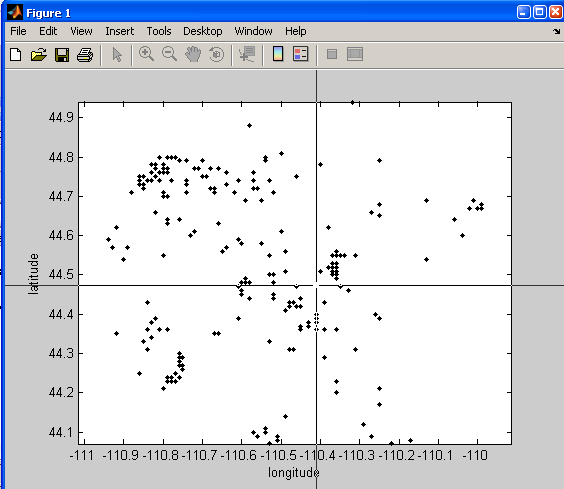The result in that case is:
```click on lower left and upper right of the area of interest
xmin =

-110.4103

xmax =

-110.2831

ymin =

44.4732

ymax =

44.5826
```

Let's draw a red box then around that selection:
```%this draws a box. Realize that it has 5 vertices because you have to close it back around to the beginning
xs = [xmin xmax xmax xmin xmin];
ys = [ymin ymin ymax ymax ymin];

hold on
plot(xs, ys, 'r-')
```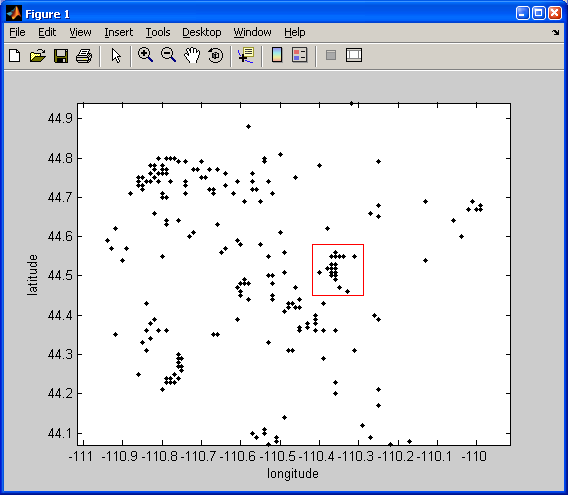#### Finding the events within a user-defined box

Now for some real interactivity. Find the events inside that square:

```t> tf = x_locations <= xmax & x_locations >= xmin & y_locations <= ymax & y_locations >= ymin;
locs = find(tf);
plot(x_locations(locs),y_locations(locs),'ro')
```
Video example:

And, let me teach you about another useful interactive item: Matlab command gtext. This will let us label our box:

NOTE: input is a function that lets you write out a message or question to the screen and ask the user for input. The user can then type anything, and that input is then assigned to the variable specified by input. If you want to get really fancy, tryout the function inputdlg. This gives you a popup box to write your response.
```your_label = input('What is your label for that selection? \n', 's');

gtext(your_label, 'Color', 'r')
```
I typed
`swarm`
as the label at the command line.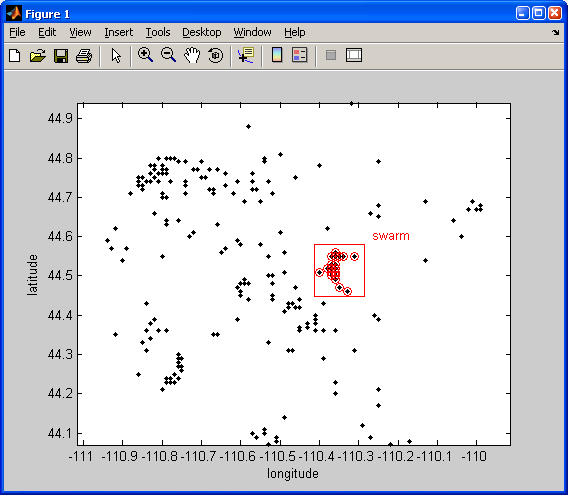#### Histograms of event year

```figure(2)
clf
years_for_hist = unique(year(locs));

subplot(2,1,1)
hist(year(locs), years_for_hist);
xlabel('Event year')
ylabel('# events')
subplot(2,1,2)
n = hist(year(locs), years_for_hist);
bar(years_for_hist, n)
xlabel('Event year')
ylabel('# events')
```
Here I have introduced the concept of subplot. By calling subplot we can place multiple axes into the same figure window and specify where in the window we want the axes to be plotted.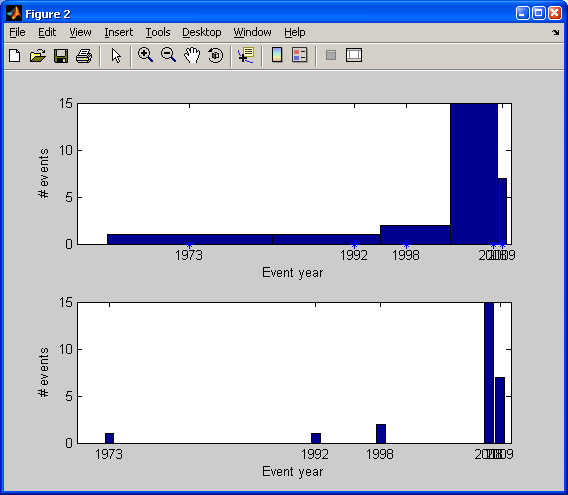Which of the above did you like better? Do you see what was different?

#### Histograms of event magnitude

```figure(3)
clf
hist(magnitudes(locs))
xlabel('Magnitude')
ylabel('# events')
```